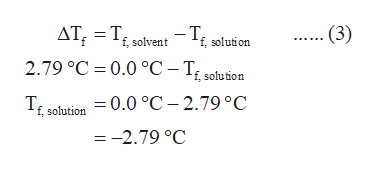# Calculate the freezing point of 1.5 moles of C6H12O6 dissolved in 1kg of water?

Question
25 views

Calculate the freezing point of 1.5 moles of C6H12O6 dissolved in 1kg of water?

check_circle

Step 1

The molality of the given solution is calculated using equation (1). In this equation m represents the molality of the solution, n represents the number of moles of solute and msolvent represents the mass of the solvent in kg.

Step 2

The van’t Hoff factor of C6H12O6 is 1 because it does not dissociate in the aqueous solution. It exists in the molecular form in the aqueous solution.

The depression in the freezing point of the given solution is calculated using equation (2). In this equation, m represents the molality of the solution, Tf represents the depression in the freezing point of the solution, Kf represents the cryoscopic constant of the solvent, and i represents the van’t Hoff factor of the solute.

Step 3

The freezing point of the given solution is calculated from the equation (3). In this equation, Tf, solution represents the...help_outlineImage TranscriptioncloseΔΤ, -Τ .(3) -T f, solvent solution 2.79 °C 0.0 °C-T£ sofution T solution = 0.0 °C-2.79 °C --2.79 °C fullscreen

### Want to see the full answer?

See Solution

#### Want to see this answer and more?

Solutions are written by subject experts who are available 24/7. Questions are typically answered within 1 hour.*

See Solution
*Response times may vary by subject and question.
Tagged in

### Chemistry